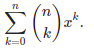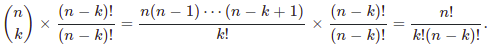# Binomial Coefficients

## Introduction-

This article will introduce Binomial Coefficients and provide approaches to use Binomial Coefficients in different data structure problems. Let’s first understand what exactly a Binomial Coefficient is?

Binomial coefficients are the number of ways to select ‘K’ items from ‘N’ different items without considering the order of arrangement of these items.

Binomial Coefficient is represented as C(N, K).

For example, there are seven balls in one box, and you have to pick three balls out of it. We can use the Binomial coefficient in this case. Here, ‘N’ is 7, and ‘K’ is 3.

The binomial Coefficient formula of calculating C(n, k) is below -

C(N, K) = C(N - 1, K - 1) + C(N - 1, K)

C(N, 0) = C(N, N) = 1

If ‘K’ = 0, it means we have to pick 0 items out of ‘N’ items and there is only one way to pick it.

Similarly, if ‘K’ = ‘N’, it means we have to pick ‘N’ items out of ‘N’ items and there is only one way to pick it.

That’s why for C(N, 0) =  C(N, N) = 1

For ‘K’ other than 0 or ‘K’, there are 2 ways to pick ‘K’ items out of ‘N’ items:

• we pick the ‘Nth’ item and then pick ‘K - 1‘ items from ‘N - 1’ items.
• we don’t pick ‘Nth’ item and go for ‘N - 1’ items and pick ‘K’ things from the rest of the ‘N - 1’ items.

This leads to the above recursive formula

Mathematical Formula -

C(N, K) = N! / K! (N - K)!

This is a standard formula of mathematics that says no of ways to pick K items out N items is represented as ‘NCK’.

Its formula is factorial of N / factorial of K * factorial of (N - K).

Example:

C(7, 3) = (7!) / (3! * 4!) = 35

So there are 35 ways to pick two balls out of seven balls, that’s how we can use the binomial coefficient concept to solve these kinds of problems.

## Brute Force Approach -

As seen above, the Formula to calculate Binomial Coefficient is a recursive formula, and we can use recursion to solve this problem.

## Algorithm -

Step 1. Create a recursive function that will accept ‘N’ and ‘K’ variables.

Step 2. Base case will be if K = 0 or K = N, then returns 1.

Step 3. Return the sum of steps 4 and 5 output.

Step 4. Recursively call the same function for ‘N - 1’ and ‘K - 1’.

Step 5. Recursively call the same function for ‘N - 1’ and ‘K’.

Algorithm Complexity:

Time Complexity: O(2 ^ (N / 2))

Recursive function ‘calculateBinomialCoefficient()’ is calling itself 2 times in every call that means recursive calls are increasing by 2 in every recursive function execution and it will keep on calling itself until ‘K’ reaches 0.

In the worst case, the value of 'K' would be 'N / 2'.

In this way, total calls will be ‘2 ^ K’ where ‘K <= N / 2’ .

Space Complexity: O(N / 2)

Internal Stack is used in recursion, and max-height could be ‘K’, i.e., 'N / 2'.

## Better Approach 1 -

The above approach is not efficient because we are solving the same problem multiple times. If we look into the recursive function closely, we can see for the same n and k, and the recursive function is being called again and again, which leads to its polynomial complexity. If the value of n is large, it would be a big problem, and the above algorithm will run forever to give the result.

What if we can store the result in some array and check-in that array? If data is present, then we will be returning it else we will calculate it?

In this case, we would be able to save a lot of computation time.

Let’s check the algorithm steps -

## Algorithm -

Step 1. Create a temp array of size ‘N + 1’ and ‘K + 1’ and initialize it with -1.

Step 2. Create a recursive function that will accept ‘N’ and ‘K’ and array.

Step 3. Base case will be if K = 0 or K = N, then returns 1.

Step 4. Check if array value for current ‘N’ and ‘K’ is other than -1, then return it.

Step 5. Recursively call the same function for N - 1 and K - 1.

Step 6. Recursively call the same function for N - 1 and K - 1.

Step 7. Save results of Steps 5 and 6 in an array for current ‘N’ and ‘K’.

Step 8. Return the result.

## Java Solution

Algorithm Complexity:

Time Complexity: O(N * K)

In this case, the recursive function ‘calculateBinomialCoefficient()’ is called for max ‘N * K’ times, which leads to O(N * K) complexity, i.e., polynomial complexity.

Space Complexity: O(N * K)

2D array of size ‘N * K’ is used to store transient results. Hence, the Space Complexity for the above approach will be O(N * K)

## Better Approach 2 -

There is an overhead of the internal stack while calling a recursive function. We can eliminate this overhead by using a non-recursive approach.

We will be calculating results from bottom to top, populate the array, and ultimately return the top result.

## Algorithm -

Step 1. Create a ‘temp’ array of size ‘N + 1’ and ‘K + 1’ and initialize it with -1.

Step 2Now let's take an iterator pointer 'i' which will be traversing from 0 to ‘N'.

Step 3. Write an inner For loop for traversing index ‘j’ from 0 to min(i, n).

Step 4. If the value of the iterator of the inner loop i.e., ‘j’ is 0 or 1 then the value of   tempArray[i][j] is 1.

Step 5. Else calculate the result from previously stored values from tempArray[i - 1][j - 1], and tempArray[i - 1][j].

Step 6. Return result from the data present at index tempArray[n][k] in the array.

## Java Solution

Algorithm Complexity:

Time Complexity: O(N * K)

This function ‘calculateBinomialCoefficient()’ would be called for max ’N * K’ times, which leads to O(N * K) complexity, i.e., polynomial complexity.

Space Complexity: O(N * K)

2D array of size ‘N * K‘ is used to store transient results. Hence, the Space Complexity for the above approach will be O(N * K)

## Approach 3-

We can avoid using a 2D array and instead we can use 1D array of size k to store temporary results. In this way, our space complexity will drastically reduce from ‘N * K’ to ‘K’.

## Algorithm -

Step 1. Create a temp array of size ‘K + 1’.

Step 2. Write outer For loop for traversing index ‘i’ from  0 to ‘N’.

Step 3. Write an inner For loop for traversing index ‘j’ from 0 to min(i, n).

Step 5. Calculate the result from previously stored values from tempArray[j - 1] and tempArray[j].

Step 6. Return result from the data present tempArray[K] in the array.

## Java Solution

Algorithm Complexity:

Time Complexity: O(N * K)

In this case, this function ‘calculateBinomialCoefficient()’ would be called for max ‘N * K’ times, which leads to O(N * K) complexity, i.e., polynomial complexity.

Space Complexity: O(k)

1D array of size ‘K’ is used to store transient results. Hence, the Space Complexity for the above approach will be O(K)

## Optimal Approach -

We can optimize the time and space complexity by simply the C(N, K) formula.

C(N, K) = N! / (N - K)! * K!

= ( [N * (N - 1) *....* 1] )  / [ ( (N - K) * (N - K - 1) * .... * 1) * ( K * (K - 1) * .... * 1 ) ]

This can be written as :

C(N, K) = [N * (N - 1) * .... * (N - K + 1)] / [K * (K - 1) * .... * 1]

Also, we can write C(N, K) = C(N, N - K) as ‘K’ can be changed to ‘N - K’

if K > (N - K)

Using the above simplification, let’s write the algorithm.

## Algorithm -

Step 1. Initialize a variable result with 1.

Step 2. If K > N - K then change ‘K’ to ‘N -  K’.

Step 3. Run a for loop with index ‘i’ from 0 to ‘K’ and execute steps 5 and 6 in that loop.

Step 5. Multiply the result by N - i.

Step 6. Divide the result by ‘i + 1’.

Step 7. Return the result.

## Java Solution

Algorithm Complexity:

Time Complexity: O(K)

As we are iterating our loop for ‘K’ times only, therefore, the overall time complexity is O(K).

Space Complexity: O(1)

As we are not using any extra space so the overall space complexity is O(1).

1) What is a Binomial Coefficient?

Binomial coefficients are the number of ways to select ‘K’ items from ‘N’ different items without considering the order of arrangement of these items.

It is represented as C(N, K).

2) What is the Formula to calculate Binomial Coefficient?

Binomial Coefficient can be calculated using the below formula-

C(n, k) = C(n-1, k-1) + C(n-1, k)

C(n, 0) = C(n, n) = 1

Mathematical Formula -

C(n, k) = n! / k! (n-k)!

3) How is the Factorial Formula to calculate Binomial Coefficient derived?

The binomial theorem expansion of ‘(1 + X) ^ N’ is called the Maclaurin series of ‘(1 + X) ^ N’. The binomial coefficient is the coefficient of ‘X ^ K’ in the series. Let’s denote the coefficient by ‘nCk,’ and therefore‘f(x) = (1 + X) ^ N’ , which is equal to

Now multiplying ‘nCk’ with ‘(n - k)! / (n - k)!’ which equals 1.## Key takeaways-

In this article, we discussed the Binomial Coefficient and the different ways possible to calculate it programmatically. We understand how we can reduce time and space complexities from exponential to polynomial using extra space. If you want to practice more problems, then you can visit codestudio.

If you think that this blog helped you, then share it with your friends!. Refer to the DSA C++ course for more information.

Until then, All the best for your future endeavours, and Keep Coding.By Harsh Goyal